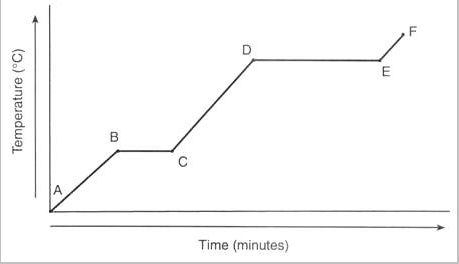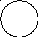# Unit 1: Getting Ready for Chemistry

 Page 2/19 Date 23.04.2018 Size 1.3 Mb. #45980

 _____12. I can describe the trend in solubility for gases as the temperature changes. As the temperature increases, the solubility of a gas ___decreases______. _____13. I can use Reference Table F to determine if a substance will be soluble in water. Write “S” for soluble and “NS” for not soluble. Use Reference Table F to determine the solubility of the following compounds: ___S__potassium chlorate ___NS__silver bromide __S___lithium carbonate __NS__calcium carbonate _____14. I can use Table G to determine how much solute to add at a given temperature to make a saturated solution. How many grams of KClO3 must be dissolved in 100 grams of water at 20oC to make a saturated solution? 56 g _____15. I can use Table G to determine if a solution is saturated, unsaturated, or supersatured. If 20.0 g of NaNO3 are dissolved in 100.0 g of water at 25.0oC, will the resulting solution be saturated, unsaturated, or supersaturated? unsaturated _____16. I can define: dilute, concentrated, concentration, and electrolyte. Definitions: dilute – a solution in which a small quantity of solute is dissolved in a large quantity of solvent concentrated – a solution in which a large quantity of solute is dissolved in a small quantity of solvent concentration – the amount of solute dissolved in a given amount of solvent electrolyte – a substance that can conduct electricity when dissolved in water _____17. I can interpret Table G to determine which solution is the most concentrated or the most dilute. Which solution is most concentrated? A) 125.0 g of KI dissolved in 100.0 g of water at 10oC B) 70.0 g of NH4Cl dissolved in 100.0 g of water at 70oC C) 120.0 g of KNO3 dissolved in 100.0 g of water at 70oC D) 30.0 g of SO2 dissolved in 100.0 g of water at 90oC _____18. I can use Reference Table T to calculate the concentration of a solution in ppm. What is the concentration, in ppm, of a 2600 g of solution containing 0.015 g of CO2? 5.7 ppm _____19. I can use Reference Table T to calculate the concentration of a solution in molarity. What is the molarity of 3.5 moles of NaBr dissolved in 500 mL of water? 7M

 _____20. I can determine how matter will be separated using filtration. When a mixture of sand, salt, sugar, and water is filtered, what passes through the filter? salt, sugar, and water _____21. I can describe how matter can be separated using distillation. Which physical property makes it possible to separate the components of crude oil by means of distillation? difference in boiling points _____23. I can state which separation process (decanting, filtering, distilling, chromatography, or evaporating) is best for a given situation. To separate a mixture of salt and water, the best method of separation would be_evaporation_. To separate a mixture of ethanol and water, the best method of separation would be ___distillation____. To separate a mixture of food coloring dyes, the best method of separation would be _____chromatography____. To separate a mixture of oil and water, the best method of separation would be_______decanting____. _____24. I can define allotrope. Defintion: allotrope – different forms of the same element that possess different molecular structures _____25. I can state the differences between two allotropes of the same element. Two allotropes of the same element have different molecular structures and therefore have different _physical___ and ___chemical_____ properties.

Unit 3: Matter & Energy

If you can do all the things listed below, you are ready for the Unit 3 test.

Place a checkmark next to each item that you can do! If a sample problem is given, complete it as evidence.

_____1. I can still do everything from Unit 1.

_____2. I can still do everything from Unit 2.

_____3. I can define kinetic energy, potential energy, temperature, heat, endothermic, and exothermic.

Defintions:

kinetic energy – energy a substance has due to its motion

potential energy – energy a substance has that is stored in chemical bonds and static interactions

temperature – a measure of the average kinetic energy of a substance

heat – energy the moves between two substances due to differences in temperature between the substances

endothermic – chemical reaction or physical change that requires the input of energy to occur

exothermic – chemical reaction of physical change that releases energy as it occurs

____4. I can use particle diagrams to show the arrangement and spacing of atoms/molecules in different phases.

Draw a particle diagram to represent atoms of Li in each phase.

 Solid Liquid Gas

_____5. I can compare solids, liquids, and gases in terms of their relative kinetic energy, type of molecular motion, ability to completely fill a container, ability to change shape.
 Solid Liquid Gas Relative Kinetic Energy low moderate high Type of Molecular Motion vibrations, only vibration and rotation vibration, rotation, and translation Ability to Completely Fill Any Container no no yes Ability to Change Shape no yes yes

_____6. I can state the change of phase occurring in fusion, solidification, condensation, vaporization, melting, boiling, sublimation, deposition, and freezing.

During fusion a substance changes from _solid___ to __liquid___.

During solidification a substance changes from _____liquid_____ to ____solid_____.

During condensation a substance changes from ____gas______ to ___liquid____.

During vaporization a substance changes from ___liquid_____ to ___gas________.

During melting a substance changes from ______solid_____ to ____liquid_______.

During boiling a substance changes from ____liquid_______ to ____gas______.

During sublimation a substance changes from ___solid_____ to ____gas______.

During deposition a substance changes from ____gas______ to ___solid_______.

During freezing a substance changes from _____liquid____ to ___solid_________.

_____7. I can indicate if a phase change is exothermic or endothermic.

For each phase change listed, indicate whether the change is exothermic or endothermic.

fusion/melting___ENDO______

solidification/freezing_______EXO__________
condensation______EXO_________
vaporization/boiling_____ENDO____________
sublimation_______ENDO__________
deposition________EXO_______________

_____8. Given a heating/cooling curve, I can determine the temperature at which a substance freezes/melts or condenses/vaporizes.

113oC

53oCWhat is the freezing point of this substance? 53oC

What is the boiling point of this substance? 113oC

_____9. Given a heating/cooling curve, I can determine which sections of the curve show changes in potential energy.On the graph, circle the sections that show a change in potential energy.

_____10. Given a heating/cooling curve, I can determine which sections of the curve show changes in kinetic energy.On the graph, circle the sections that show a change in kinetic energy.

_____11. I can state the temperature at which water freezes in oC and K.

What is the freezing point of water in oC and K?
0oC and 273K

_____12. I can state the temperature at which water melts in oC and K.

What is the melting point of water in oC and K?
0oC and 273K

_____13. I can state the temperature at which water vaporizes/boils in oC and K.

What is the boiling point of water in oC and K?
100oC and 373K

_____14. I can state the temperature at which water condenses in oC and K.

What is the condensing point of water in oC and K?
100oC and 373K

 _____15. I can use Reference Table T to determine which “heat” equation is needed for a given problem. Which heat equation should be used in each of the following: a. How much heat is needed to vaporize 100.0 g of water at 100oC? Q = mHv b. How much heat is needed to raise the temperature of 100.0 g of water by 35oC? Q=mCT c. How much heat is needed to melt 100.0 g of ice at 0oC? Q = mHf _____16. I can define specific heat capacity, heat of fusion, heat of vaporization. Definitions: specific heat capacity – the amount of heat required to increase the temperature of one gram of substance by 1oC (or K) heat of fusion - the amount of heat required to melt one gram of substance at its melting point heat of vaporization - the amount of heat required to vaporize one gram of substance at its boiling point _____17. I can use the “heat” equations to solve for any variable, if I am given the other variables. How many grams of water can be heated by 15.0oC using 13,500 J of heat? 215 g It takes 5210 J of heat to melt 50.0 g of ethanol at its melting point. What is the heat of fusion of ethanol? 104 J/g _____18. I can state the 5 parts of the Kinetic Molecular Theory. The five parts of the Kinetic Molecular Theory are: a. Gases consist of tiny particles. b. The size of the particles is so small compared to the space between the particles that the volume of the actual gas particles is negligible. c. Gas particles are in constant, random, straight-line motion, colliding with the walls of the container. These collisions create pressure. d. Gas particles have no intermolecular forces (IMF). e. The average kinetic energy of gas particles is directly proportional to their Kelvin temperature. _____19. I can define an ideal gas. Definition: ideal gas –any gas that conforms to all of the parts of the KMT. Ideal gases are theoretical although some gases are close. Hydrogen and helium are the closest to ideal gases at all temperatures and pressures. _____20. I can state the conditions of pressure and temperature under which a gas will act “ideally”. A gas will act most “ideally” under the conditions of __low___ pressure and ___high_____ temperature. _____21. I can state the two elements that act ideally most of the time. The two elements that act ideally most of the time are ___hydrogen______ & _____helium_______. _____22. I can explain how pressure is created by a gas. What causes gas molecules to create pressure? Collisions with the walls of the container. _____23. I can state the relationship between pressure and volume for gases (assuming constant temperature). At constant temperature, as the pressure on a gas increases, the volume ____decreases______. _____24. I can state the relationship between temperature and volume for gases (assuming constant pressure). At constant pressure, as the temperature on a gas increases, the volume _____increases_____. _____25. I can state the relationship between temperature and pressure for gases (assuming constant volume). In a fixed container (AKA “has constant volume), as the temperature on a gas increases, the pressure____increases______. _____26. I can state Avogadro’s Hypothesis. Avogadro’s Hypothesis says two samples of an ideal gas, if they have the same temperature, pressure, and volume, will contain the same number of molecules. _____27. I can remember to convert oC to K when using the Combined Gas Law to determine changes in V, P, or T of a gas. A gas originally occupies 2.3L at 56oC and 101.3 kPa. What will its volume be at 100oC and 105.7 kPa? 2.5 L

 _____28. I can define boiling point and vapor pressure. Definition: boiling point – the temperature at which the vapor pressure of a liquid equals the pressure surrounding the liquid vapor pressure – the pressure exerted by a vapor in equilibrium with its condensed phases (solid or liquid) at a given temperature in a closed system _____29. I can state the condition of pressure that is used for “normal” boiling points. The normal boiling point of a substance occurs at a pressure of ___1______atm/_____101.3_____kPa. _____30. I can state the relationship between atmospheric pressure and boiling point. As the atmospheric pressure increases, the boiling point ___increases_____.